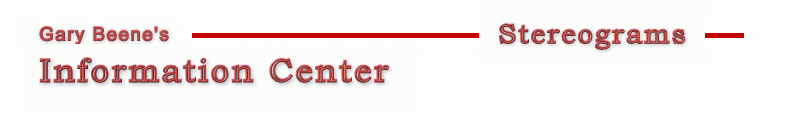Stereogram Algorithms - Basic It takes surprisingly few lines of code to generate a stereogram. The 25 lines of code below will create a sterogram. Stereograms created by this minimal code are fun to view, but suffer from several visual shortcomings - primarily the apparent layering of the object being viewed. Other visual issues (echoes, artifacts, holes, ...) can also be seen in the results of the basic code. Fortunately, improvements in the basic algorithm are available which can significantly improve the resulting stereograms. These algorithm improvements, and the source code to implement them, are discussed on other pages (see the menu links under Algorithm on the left side of this page). Smart Links Hidden Surface Removal Oversampling Center-out Processing Basic Stereogram Source Code In this example, two pictureboxes are used - one to provide the depth information (picDepth) and one to display the stereogram (picOut). ```1 Dim x As Long, y As Long, maxX As Long, maxY As Long, same() As Long 'basic 2 Dim z() As Single, mu As Single, E As Long, DPI As Long, s As Long 'basic 3 Dim right As Long, left As Long, w As Single 'basic 4 DPI = 72: E = 2.5 * DPI: mu = 0.2: w = 0.5 5 maxX = picDepth.ScaleWidth: maxY = picDepth.ScaleHeight 6 ReDim same(maxX), z(maxX, maxY): Randomize 7 For y = 0 To maxY 8 For x = 0 To maxX - 1 '1 scanline 9 z(x, y) = picDepth.Point(x, y) / 16777215 'initialize 10 same(x) = x 'initialize 11 s = (1 - mu * z(x, y)) * E / (2 - mu * z(x, y)) 'image plane separation 12 left = x - (s / 2) 'left link position 13 right = left + s 'right link position 14 If 0 <= left And right < maxX Then same(left) = right 'create link 15 Next x 16 For x = maxX - 1 To 0 Step -1 'set colors 17 If same(x) = x Then 18 picOut.PSet (x, y), Int(Rnd + w) * vbWhite 'random Rnd*vbwhite for color 19 Else 20 picOut.PSet (x, y), picOut.Point(same(x), y) 'use link color 21 End If 22 Next x 23 Next y ``` Code Discussion The code works in the following way ...